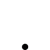# 找尋離本日最近的紅(黑)棒

•   76
• 最後發表   隔壁老王  2022 一月 27

GammaCEO 發文於   2022/01/18

``` //前黑高
if BarsLast(close<open)=1 or close<open then
value1=high;

plot1(value1,"前黑高");```

Value1 = Average(close,5);

Value2 = Average(close,20);

if BarsLast(close<open)=1 or close<open then

value3=high;

if c > o

and c > Value1

and c > Value2

and c > Value3

then ret=1;

XQ小幫手 發文於   2022/01/24

Hello 隔壁老王,

value1 = Average(close,5);

value2 = Average(close,20);

value3 = high[barslast(open > close)];

condition1 = close > open;

condition2 = close > value1;

condition3 = close > value2;

condition4 = close > value3;

if condition1 and condition2 and condition3 and condition4 then ret = 1;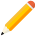發表新主題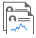我的發文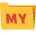我的收藏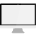分類

Close